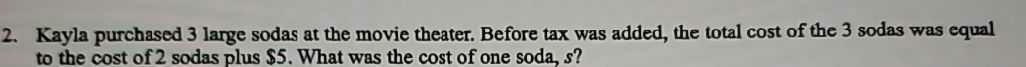### ¿Todavía tienes preguntas de matemáticas?

Pregunte a nuestros tutores expertos
Algebra
Pregunta2. Kayla purchased $$3$$ large sodas at the movie theater. Before tax was added, the total cost of the $$3$$ sodas was equal to the cost of $$2$$ sodas plus $$\ 5$$ . What was the cost of one soda, $$s$$ ?